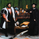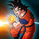2379 görüntülenme
This is the study with allerts of the RSI-MTF2

if there is repainting then I suggest to go to lower time frame and graph of 1 hour or half hour then it will not repaint. the issue i think exist only in 24 hours time frame when you build it on shorter graph
still the results will be great (test it in strategy that i put before this one)

have fun

## YorumlarAs a side note. I found that in lower TFs your strat sometimes raises false flags and i found the reason (probably).
Use variance(price, lookback) as a filter.
When the signal is fired and variance is below some threshold- wait for the rise of the variance and enter in the direction of the signal.
Cevap GönderRafaelZioni
@RafaelZioni,
X = input(hlc3)
p = input(2)
mult = input(1., minval=.01, step=.1)
mean(x, p) => sum(x, p) / p
mean_price = mean(X, p) // OR sma et al.
var = variance(mean_price, p)
iv = inverse_fisher(var*100) * mult // this is the case for BTCUSD. Ex. in EUR the variance is very small, so stddev is better. To be tested.
plot(iv, transp=0)
Cevap GönderPosted a rebrush of your code here

also variance as a filter may be used this way:
buy = crossover(value, threshold2) and var > thres_var
sell = crossunder(value, threshold1) and var > thres_var
Cevap GönderRafaelZioni
@RafaelZioni, I set the MTF in the script to 180 min and my chart on TV for 30 min, is this correct? thanks for the script it looks very promising
Cevap Gönder